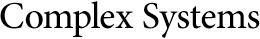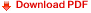## Some Quantum Mechanical Properties of the Wolfram ModelJonathan Gorard
University of Cambridge
jg865@cam.ac.uk
and
Wolfram Research, Inc.
jonathang@wolfram.com

#### Abstract

This paper builds upon the techniques developed within our previous investigation of the relativistic and gravitational properties of the Wolfram Model—a new discrete spacetime formalism based on hypergraph transformation dynamics—in order to study classes of such models in which causal invariance is explicitly violated, as a consequence of non-confluence of the underlying rewriting system. We show that the evolution of the resulting multiway system, which effectively contains all possible branches of evolution history (corresponding to all possible hypergraph updating orders), is analogous to the evolution of a linear superposition of pure quantum eigenstates; observers may then impose “effective” causal invariance by performing a Knuth–Bendix completion operation on this evolution, thus collapsing distinct multiway branches down to a single, unambiguous thread of time, in a manner analogous to the processes of decoherence and wavefunction collapse in conventional quantum mechanics (and which we prove is compatible with a multiway analog of the uncertainty principle). By defining the observer mathematically as a discrete hypersurface foliation of the multiway evolution graph, we demonstrate how this novel interpretation of quantum mechanics follows from a generalized analog of general relativity in the multiway causal graph, with the Fubini–Study metric tensor playing the role of the spacetime metric, the quantum Zeno effect playing the role of gravitational time dilation and so on. We rigorously justify this correspondence by proving (using various combinatorial and order-theoretic techniques) that the geometry of the multiway evolution graph converges to that of complex projective Hilbert space in the continuum limit, and proceed to use this information to derive the analog of the Einstein field equations for the overall multiway system. Finally, we discuss various consequences of this “multiway relativity,” including the derivation of the path integral, the derivation of particle-like excitations and their dynamics, the proof of compatibility with Bell’s theorem and violation of the CHSH inequality, the derivation of the discrete Schrödinger equation, and the derivation of the nonrelativistic propagator. Connections to many fields of mathematics and physics—including mathematical logic, abstract rewriting theory, automated theorem-proving, universal algebra, computational group theory, quantum information theory, projective geometry, order theory, lattice theory, algorithmic complexity theory, advanced combinatorics, superrelativity, twistor theory and AdS/CFT-correspondence—are also discussed.

Cite this publication as:
J. Gorard, “Some Quantum Mechanical Properties of the Wolfram Model,” Complex Systems, 29(2), 2020 pp. 537–598.
https://doi.org/10.25088/ComplexSystems.29.2.537